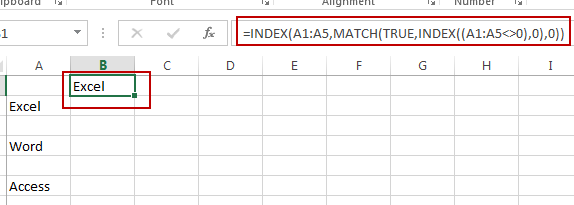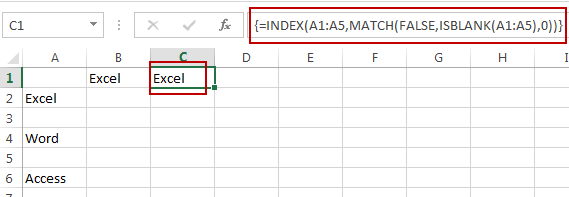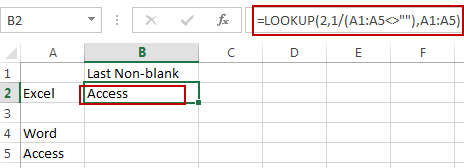# How to Find the First or Last Non-blank Cell in a Row or Column in Excel

This post will guide you how to find the first non-blank cell in a row or column or a range in Excel. How do I get the value of the last non-blank cell in a row or column using formula in Excel.

## Get First Non-blank Cell Value

Assuming that you have a list of data in range A1:A5, and you want to find the first non-blank cell in a given range of cells in Excel. How to do it. You can use a formula based on the INDEX function and the MATCH function to get the first non-blank value in a one-column range in Excel. Here is the formula you can use:

`=INDEX(A1:A5,MATCH(TRUE,INDEX((A1:A5<>0),0),0))`

Type this formula into a blank cell and press Enter key on your keyboard. You would get the first non-blank cell value from your given range A1:A5.Or you can use an array formula based on the INDEX function, the MATCH function and the ISBLANK function to achieve the same result of extracting the first non-blank cell value from a given range in Excel. Like this:

`=INDEX(A1:A5,MATCH(FALSE,ISBLANK(A1:A5),0))`

Type this formula into a blank cell and press Ctrl + Shift + Enter keys on your keyboard to make your formula as array formula.## Get Last Non-blank Cell Value

If you want to find the value of the last non-blank cell in a row or column in Excel, you can use a formula based on the LOOKUP function to achieve it. Here is the formula we are using is:

`=LOOKUP(2,1/(A1:A5<>""),A1:A5)`

Type this formula into a blank cell and press Enter key on your keyboard. You would get the last non-blank cell value from your given range A1:A5.### Related Functions

• Excel LOOKUP function
The Excel LOOKUP function will search a value in a vector or array.The syntax of the LOOKUP function is as below:= LOOKUP (lookup_value, lookup_vector, [result_vector])…
• Excel INDEX function
The Excel INDEX function returns a value from a table based on the index (row number and column number)The INDEX function is a build-in function in Microsoft Excel and it is categorized as a Lookup and Reference Function.The syntax of the INDEX function is as below:= INDEX (array, row_num,[column_num])…
• Excel MATCH  function
The Excel MATCH function search a value in an array and returns the position of that item.The MATCH function is a build-in function in Microsoft Excel and it is categorized as a Lookup and Reference Function.The syntax of the MATCH function is as below:= MATCH  (lookup_value, lookup_array, [match_type])….
• Excel ISBLANK function
The Excel ISBLANK function returns TRUE if the value is blank or null.The syntax of the ISBLANK function is as below:= ISBLANK (value)…